# Atomic Structure and Periodic TrendsPage 16

#### WATCH ALL SLIDES

d) All anions are larger than their parent atoms and all cations are smaller, compare Be2+(27 pm) and Be (112pm), I-(206pm) and I(133) – please note that ionic radius depends on coordination number of ion

e) Ionic radii generally decrease with increasing positive charge on the same ion (Tl+, 164pm > Tl3+, 88pm)

Slide 135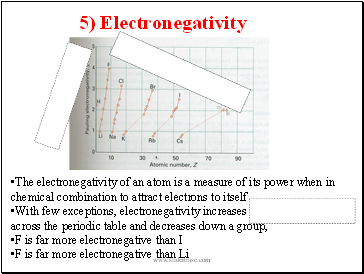5) Electronegativity

The electronegativity of an atom is a measure of its power when in chemical combination to attract electrons to itself

With few exceptions, electronegativity increases across the periodic table and decreases down a group,

F is far more electronegative than I

F is far more electronegative than Li

Slide 136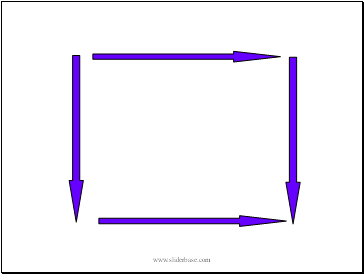Slide 137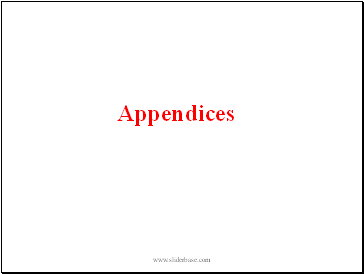## Appendices

Slide 138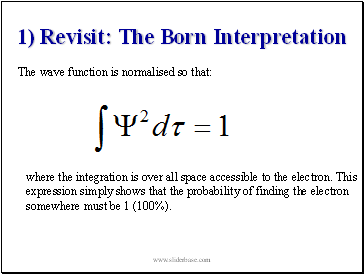1) Revisit: The Born Interpretation

The wave function is normalised so that:

where the integration is over all space accessible to the electron. This expression simply shows that the probability of finding the electron somewhere must be 1 (100%).

Slide 139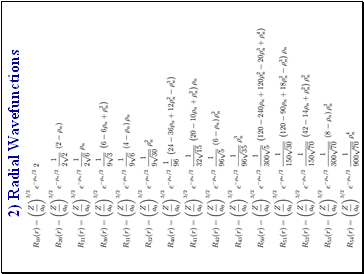Slide 140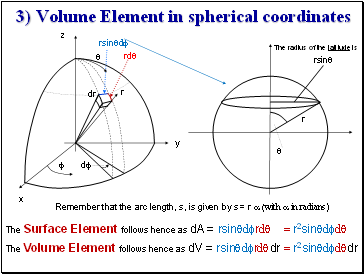r

z

y

x

dr

rdq

rsinqdf

df

f

q

q

r

rsinq

The radius of the latitude is

Remember that the arc length, s, is given by s = r a (with a in radians)

The Volume Element follows hence as dV = rsinqdfrdqdr = r2sinqdfdqdr

3) Volume Element in spherical coordinates

The Surface Element follows hence as dA = rsinqdfrdq = r2sinqdfdq

Slide 141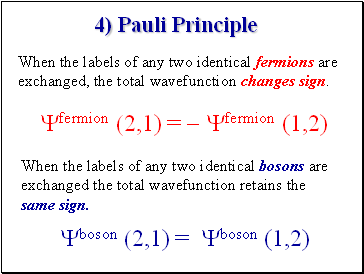4) Pauli Principle

Yfermion (2,1) = - Yfermion (1,2)

Yboson (2,1) = Yboson (1,2)

When the labels of any two identical fermions are exchanged, the total wavefunction changes sign.

When the labels of any two identical bosons are exchanged the total wavefunction retains the same sign.

Slide 142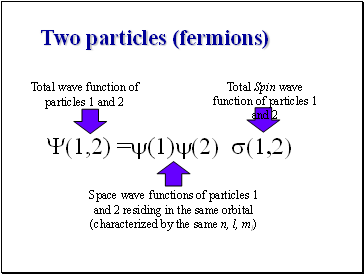Two particles (fermions)

Y(1,2) =y(1)y(2) s(1,2)

Total wave function of particles 1 and 2

Space wave functions of particles 1 and 2 residing in the same orbital (characterized by the same n, l, ml)

Total Spin wave function of particles 1 and 2

Slide 143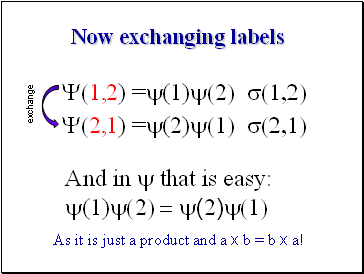Now exchanging labels

As it is just a product and a x b = b x a!

Y(1,2) =y(1)y(2) s(1,2)

And in y that is easy:

y(1)y(2) = y(2)y(1)

Go to page:
1  2  3  4  5  6  7  8  9  10  11  12  13  14  15
16  17